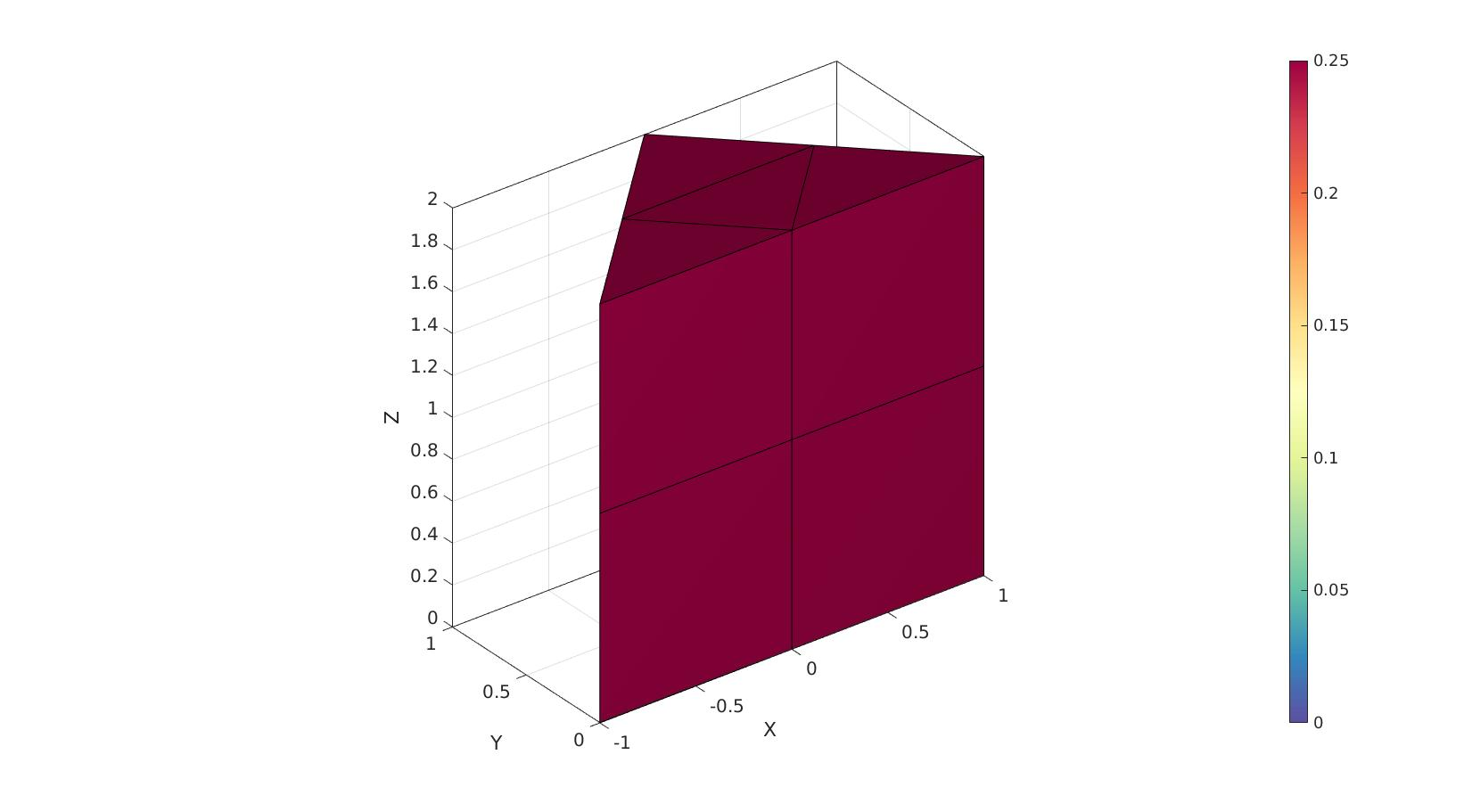# pentaVol

Below is a demonstration of the features of the pentaVol function

## Contents

```clear; close all; clc;
```

## Syntax

[VE,L]=pentaVol(E,V);

## Description

This function computes pentahedral element volumes. The input is the element description (E) and the nodes (V). The output is the element volumes (always positive) and a logic denoting wheter the element appears to be valid (1) or inverted (0).

## Examples

Plot settings

```cMap=gjet(250);
faceAlpha1=1;
faceAlpha2=0.65;
edgeColor1='none';
edgeColor2='none';
fontSize=15;
```

## Example: Computing the volume of pentahedral elements

Create example geometry

```V=[-1 0 0;...
1 0 0;...
0 1 0;...
-1 0 1;...
1 0 1;...
0 1 1;...
];

h=2;
V(:,3)=V(:,3)*h;
E=[1 2 3 4 5 6];

a=patchArea([1 2 3],V);

[E,V,C,CV]=subPenta(E,V,1,1);
```

Computing the volume

```[VE,logicPositive]=pentaVol(E,V,0);

[F,CF]=element2patch(E,VE,'penta6');

cFigure; hold on;
% gpatch(F,V,'w','none',0.25);
gpatch(F,V,CF,'k',1);
% patchNormPlot(F,V);
caxis([0 max(VE(:))]);
colormap spectral; colorbar;
drawnow;
```The summed volume should match the theoretical

```volume_theoretical=a*h;
volume_total=sum(VE);

disp(['Theoretical volume:',sprintf('%f',volume_theoretical)]);
disp(['Total volume computed:',sprintf('%f',volume_total)]);
```
```Theoretical volume:2.000000
Total volume computed:2.000000
```GIBBON www.gibboncode.org

Kevin Mattheus Moerman, [email protected]

GIBBON footer text

GIBBON: The Geometry and Image-based Bioengineering add-On. A toolbox for image segmentation, image-based modeling, meshing, and finite element analysis.

Copyright (C) 2006-2022 Kevin Mattheus Moerman and the GIBBON contributors

This program is free software: you can redistribute it and/or modify it under the terms of the GNU General Public License as published by the Free Software Foundation, either version 3 of the License, or (at your option) any later version.

This program is distributed in the hope that it will be useful, but WITHOUT ANY WARRANTY; without even the implied warranty of MERCHANTABILITY or FITNESS FOR A PARTICULAR PURPOSE. See the GNU General Public License for more details.

You should have received a copy of the GNU General Public License along with this program. If not, see http://www.gnu.org/licenses/.